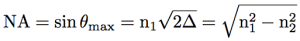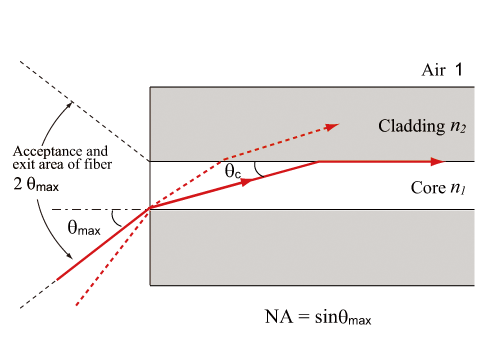Figure shows a section of optical fiber. The maximal incident angle for the total internal reflection of light in the core is called as maximum acceptance angle, θmax. The maximum acceptance angle is represented by numerical aperture (NA). Provided that the surrounding of optical fiber is the air (nair=1), by combining Snell’s law at the fiber edge face and this equation, NA is obtained as follows.Above equation indicates that NA is controlled by changing the refractive index difference. For instance, provided n1=1.4675 and n2=1.4622, NA=0.125 is obtained. In order to efficiently introduce light in the optical fiber by using lens, it is usually better that NA of the lens is equal to or lower than NA of the optical fiber.Fig. Numerical aperture of optical fiber.